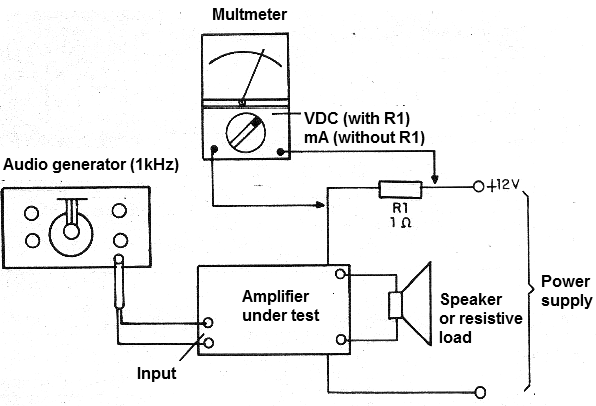## Measuring the Consumption of an Amplifier (INS042S)

This procedure allows to measure the power consumed by an amplifier at rest and also with the whole open volume, allowing the determination of its efficiency. With this measure, it will be possible to effectively determine the RMS power of an amplifier. For measurements we will need the following material:

a) Milliammeter or multimeter

b) Signal injector or audio generator (functions)

c) R1 - 1 ohm resistor x 5 W (optional)

Procedure

In the figure below we show how to make the connections of the elements of the circuit for the measurement.We can either connect the milliammeter to the appropriate current range in series with the continuous supply of the amplifier as we use the resistor and measure the voltage on it.

We initially measured the voltage on the resistor with no input signal to measure the power consumed without signal (rest). See INS122,

Then, we apply a 1 kHz signal to the amplifier input and open the entire volume.

Measure the current in the amplifier or measure the voltage across the resistor.

By multiplying the current by the supply voltage, we have the effective consumption and therefore the RMS power can be obtained by subtracting the power at rest.

By multiplying the measured voltage on the resistor by its resistance we have the power consumed. Likewise, by subtracting the power at rest we have the output RMS power.